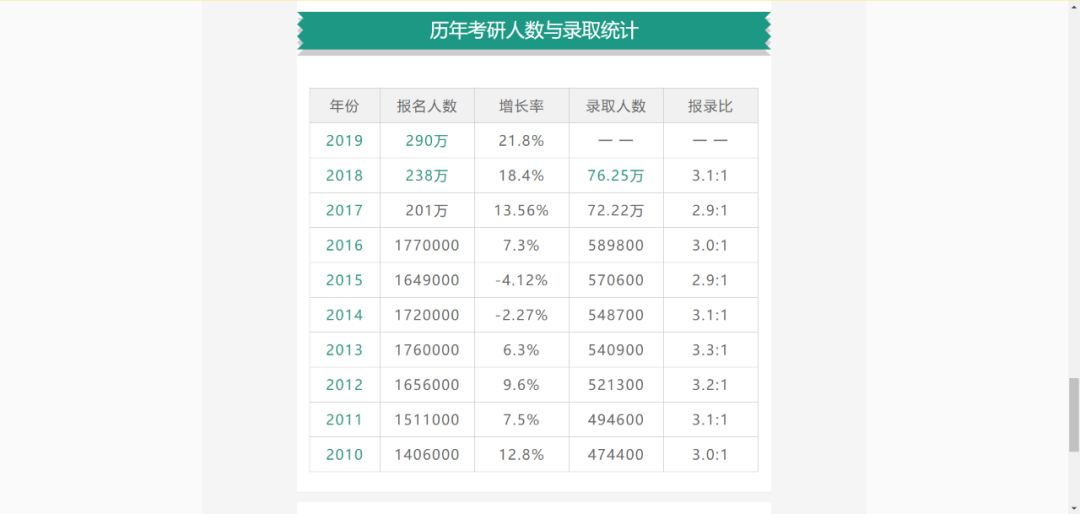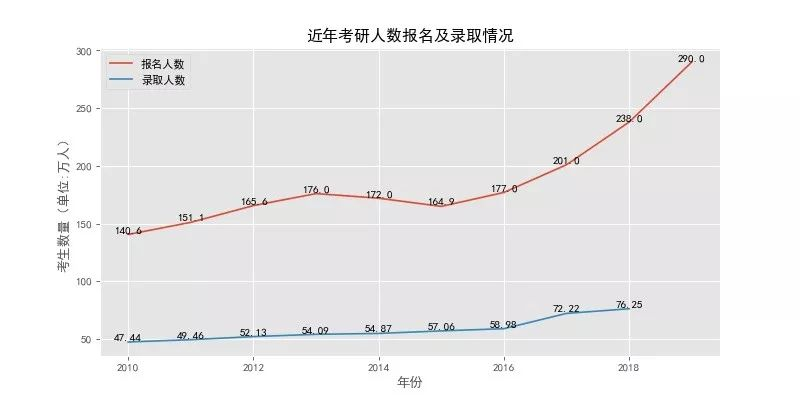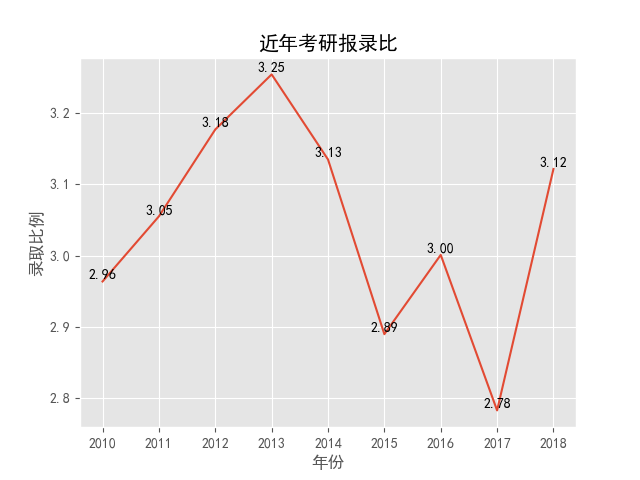# 用 Python 分析今年考研形势2017年考研人数增长至201万人，

2018年则达到238万人，

2019年考研报名人数达到290万人。import pandas as pd
import matplotlib.pyplot as plt

# 第一，读取数据
# print(df)

# 第二，绘制折线图

plt.rcParams['font.sans-serif'] = ['SimHei']
# 可以解释中文无法显示的问题

# 1）创建画布
plt.figure(figsize=(10,5),dpi=80)

# 2）绘制图像

plt.style.use('ggplot')
# matplotlib官方提供了五种不同的图形风格，
# 分别是：bmh、ggplot、dark_background、fivethirtyeight和grayscale

plt.plot(df["年份"], df["报名人数"] / 10000, label="报名人数")
plt.plot(df["年份"][:-1], df["录取人数"][:-1] / 10000, label="录取人数")

plt.title("近年考研人数报名及录取情况")
plt.xlabel("年份")
plt.ylabel("考生数量（单位:万人）")

# 设置数字标签
for a, b in zip(df["年份"], df["报名人数"] / 10000):
plt.text(a, b, b, ha='center', va='bottom', fontsize=10)

for a, b in zip(df["年份"][:-1], df["录取人数"][:-1] / 10000):
plt.text(a, b, b, ha='center', va='bottom', fontsize=10)

plt.legend()
plt.grid(True)

# 保存图像
plt.savefig("zhexian.jpg")

# 3）展示图像
plt.show()

posted @ 2020-01-15 19:16  Python学习啊  阅读(...)  评论(...编辑  收藏